Windows

# Energy Level Plot Window

This window can be used to display a wide variety of energy level plots. The plot below is a typical linear molecule plot, showing the rotational levels of the ground X 3Σ -state of SO. Plotted as a function of J, in shows the F1 F2 and F3 components as a function of J. Note that BJ(J+1) has been subtracted from the vertical scale, so that the deviations from the simple BJ(J+1) pattern are clear. The actions of the various controls are listed below.

• The status bar at the bottom will show the co-ordinates of the mouse at a minimum, and will also normally show information about calculated and observed points if over a point.
• To print the plot right click on the plot and select "Copy to clipboard" and then paste it into a suitable application. For Microsoft word, and many other applications it is important to use "paste special" rather than a simple paste (otherwise the numbers producing the plot will be pasted, rather than the plot itself).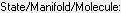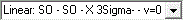Select which states to plot.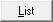Write the plotted energy levels to the Log window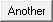Open another level Window

## Plot Type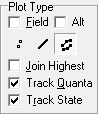The options in this group control the overall appearance of the plot.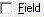Plot against external field, rather than quantum number. See Electric or Magnetic Field Plots for more details.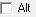Select to plot absolute symmetry, rather than J adjusted symmetry.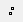Plot a symbol for each level. The key to the symbols will normally be shown at the top of the plot, and different symbols indicate different symmetries.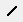Join associated levels with lines. The levels joined are controlled by the three options below.Plot both points and lines.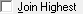Choose between starting join process from the highest energy or lowest energy pointTry to join points with related values of quantum numbersTry to join points arising from same state

## Energy Scale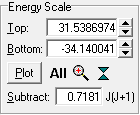The options in this group control the vertical scale of the plot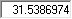Maximum energy in plot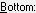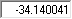Minimum energy in plotRefresh Plot (only needed if AutoReplot turned off in mixture)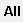Adjust plot range to include all levelsZoom to selected vertical range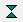Compress vertical scale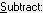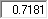Subtract number × J(J+1) from all energies plotted

## Level Selection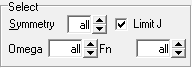This group allows levels to be selected by symmetry or some other quantum numbers. The picture is for a linear molecule; alternate quantum numbers are selected for other molecule types.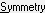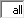Select (or not) symmetry to plotLimit J range plotted to Jmax for manifold.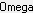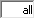Select Ω (linear molecules), Ka (asymmetric tops) |K| (symmetric tops) or v1 (Vibrating molecule).Select Fn (linear molecules), Kc (asymmetric tops) or Kl (symmetric tops).

## x Axis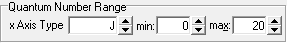For normal plots, this controls the x axis.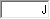Select quantum number for x axis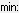Minimum J to include in plot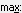Maximum J to include in plot

## Observed Level Overlay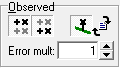It is possible to compare calculated and observed energy levels, with the "observed" energy levels calculated by combining the measured line positions with the calculated energy levels for the other state. This requires an experimental line list (a .lin file), made as described in line position fitting. To include experimental information:

1. Make sure the line list file is set in the Log window.
2. Selectto plot upper state energy levels or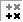to plot lower state energy levels.
3. Pressto display the observed energy levels.
4. The display is normally clearer ifis selected for the calculated energy levels.
5. If the mouse is over an experimental point it's source is displayed in the status bar; right click offers the following options:
• Edit Point: Open point under cursor in editor if possible.
• Show Line: Centre main plot on transitionPlot Upper State Observed Levels: Eobs = Elower + νobsPlot Lower State Observed Levels: Eobs = Eupper - νobs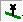Join observed point to calculated point(Re)load observations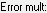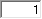When plotting observed energy levels, scale the distance from the calculated position by this. If a negative value is given, then the plotted point is Ecalc + (Eobs-Ecalc)/σ, where σ is the estimated error of the observation. If Exclude Perturbations is set, then perturbations are excluded from calculating Ecalc, but not from the Elower or Eupper value used in calculating Eobs.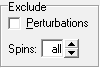This group allows the selected items to be excluded from calculations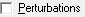Exclude perturbations in plotted levels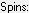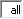Set how many nuclear and electron spins to exclude from the calculationNone LeftOut: At left edge of plot, right justified LeftIn: At left edge of plot, left justified RightIn: At right edge of plot, right justified RightOut: At right edge of plot, left justified Start: At start of the line End: At the end of the line